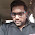### Duplicate Integer

Source: Variant of a sub-problem in my seminar presentation on "Security Attacks on RSA" . Very interesting (and difficult) algorithms problem.

Problem: An array of length n+1 is populated with integers in the range [1, n]. Find a duplicate integer (just one, not all) in linear time with O(1) space. The array is read-only and may not be modified.

Solution:
Update(20/01/10): Solution (from Gurmeet Singh Manku's blog) posted in comments!!

1.If we are given that there is exactly one element which is repeated in the array- then it is easily solvable.

I will invoke my favourite tool- xor sum of all the members of array. This sum would not contain the repeated element as a xor a=0 .

So find the xor sum of all numbers from 1 to n and further xor it with the xor sum of the elements of array. Ans would be repeated element.

But this solution is not immediately extended to case when more then one are repeated- so thinking about that !

2.@Nikhil , ur solution assumes something more : only one element is repeated EXACTLY ONCE.

with this assumption ,relatively simple solution :
Sum all elements in the array.
SUM = A+A+ ... + A[n+1]
note that SUM = n*(n+1)/2 + r , where r is repeated element.
Thus subtracting n*(n+1)/2 from SUM will give repeated element r.

3.Let p(x) be a polynomial so that coefficient of x^i is no of times (i+1) comes in the array. Given this polynomial we can obvl find all repeated elements.

But as we need to use only O(1) space- we can get this polynomial in hidden form- maintain p(n+2).

So formal soultion :
while scanning array , if you see i, add to rolling sum , (n+2)^(i-1).

Now repeatedly take mod & then divide this sum by (n+2) to recover the number of all integers appearing in the array.

PS: Only glitch- is this still O(1) space ? Sum may be very large depending on n.

4.This is not O(1). Hence not a correct solution. Interesting idea though.

A rough estimate of what your sum might be of the order of: n*n^n which would take space O(nlogn) :(

actually the naive way takes O(n) space only. So, this is not good.

5.Posting solution put on Gurmeet Singh Manku's blog:

Treat the values of the array as pointers into the same array. Let us call a chain of pointers a “linked list”. The linked list starting with the last entry must have the shape of a “lollipop” with a stick and a cycle. Our goal is to identify that node in the linked list that joins the stick to the cycle. We do so in two phases:

Phase I: maintain two pointers, one ’slow’ and one ‘fast’. In one round, the slow pointer advances one step and the fast pointer advances two steps along the linked list — both pointers advance simultaneously. Phase I comes to an end when the two pointers become equal at the end of a round.

Phase II: maintain two pointers, both ’slow’. The first slow pointer starts where Phase I ended. The second slow pointer starts at the beginning of the linked list. Phase II ends when these two pointers become equal at the end of a round. This node, magically, is the duplicate (in other words, that node in the linked list where the stick joins the cycle)!

Proof: Let s and c denote the lengths of the stick and the cycle portions of the lollipop. If the slow pointer in Phase I ends in s + x rounds, then 2(s+x) = s+x + mc, where m is a positive integer. In other words, s+x = mc. So in Phase II, by the time the second slow pointer (which starts at the node where Phase I ended) traverses s nodes, the first slow pointer traverses mc – x nodes. So both slow pointers become equal at that node where the stick attaches to the cycle.

Still hoping that someone would give a "simpler" solution.

6.I know this is an old page but I came across this and couldn't resist myself from posting a simple solution.
Take given array contains integers from 1 to n-1.
Take sum of all integers present in the array(Sa). Take sum of first n-1 natural numbers(Sn).
Sa - Sn is the duplicate entry.

7.#include

int main()
{
int dup_val=0,i;
int array[n+1]={Some n+1 values ranges from 1 to n};

for(i=1;i<=n;i++)
{
dup_val=dup_val ^ i ;
}

for(i=0;i< n+1;i++)
{
dup_val=dup_val ^ array[i] ;
}

printf("\nThe Duplicate value is %d\n\n",dup_val);

return 0;
}